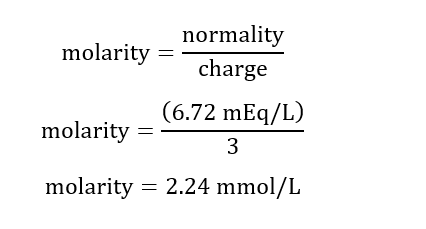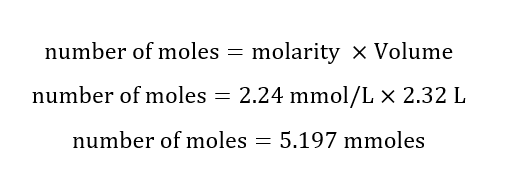# In a laboratory experiment a blood sample was found to have an iron level of 6.72 mEq/L. How many grams of iron (Fe^3+) ions are in 2.32 L of blood? Please explain each steps and show work. Thanks!

Question
1 views

In a laboratory experiment a blood sample was found to have an iron level of 6.72 mEq/L. How many grams of iron (Fe^3+) ions are in 2.32 L of blood? Please explain each steps and show work. Thanks!

check_circle

Step 1

Given:

Normality = 6.72 mEq/L

Volume of solution = 2.32 L

Charge = +3

Step 2

Calculation of molarity of blood:Step 3

Calculation for number of moles:...

### Want to see the full answer?

See Solution

#### Want to see this answer and more?

Solutions are written by subject experts who are available 24/7. Questions are typically answered within 1 hour.*

See Solution
*Response times may vary by subject and question.
Tagged in